Name: Iqbal
Level of the question: All

[tan(x)-sin(x)]/sin3(x)=sec(x)/[1+cos(x)]

Thank you

Hi Iqbal.

Remember that when you are given an equation to prove, unless you're told otherwise you can start with either side of the equation to prove the other. So pick whichever side you think is easier.

Iíll show you one way of solving your question by starting with the right hand side:

ExpressionReason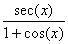Given R.H.S.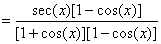Multiply by the conjugate of the denominator.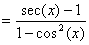Simplify.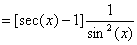Use the identity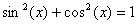and separate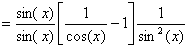Use the identity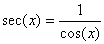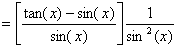Multiply the middle term through the numerator.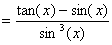As required.

Do you think it would be harder or easier starting from the left hand side? Try it!

I hope this helps you with other proofs based on trig identities, Iqbal!
Stephen La Rocque>